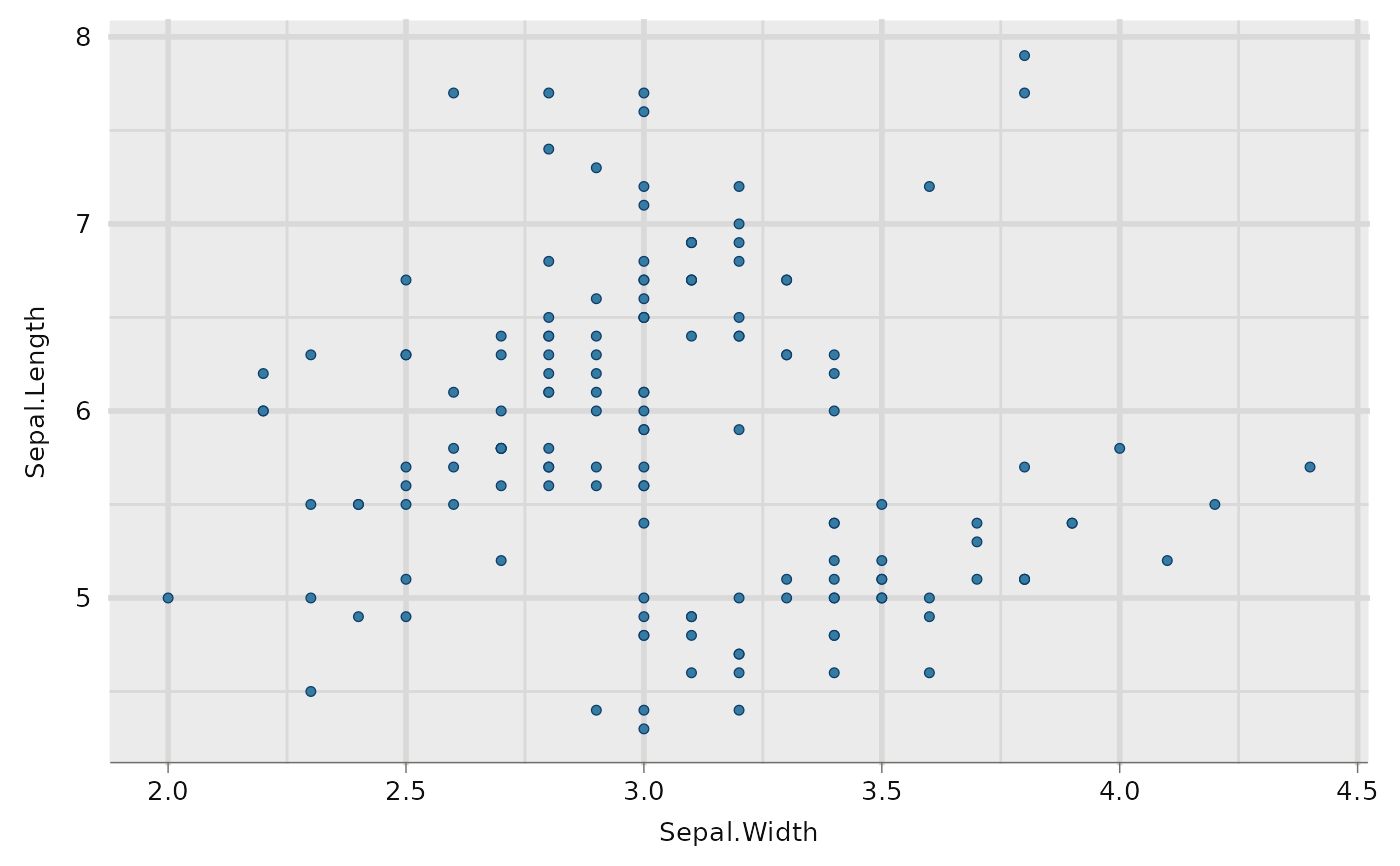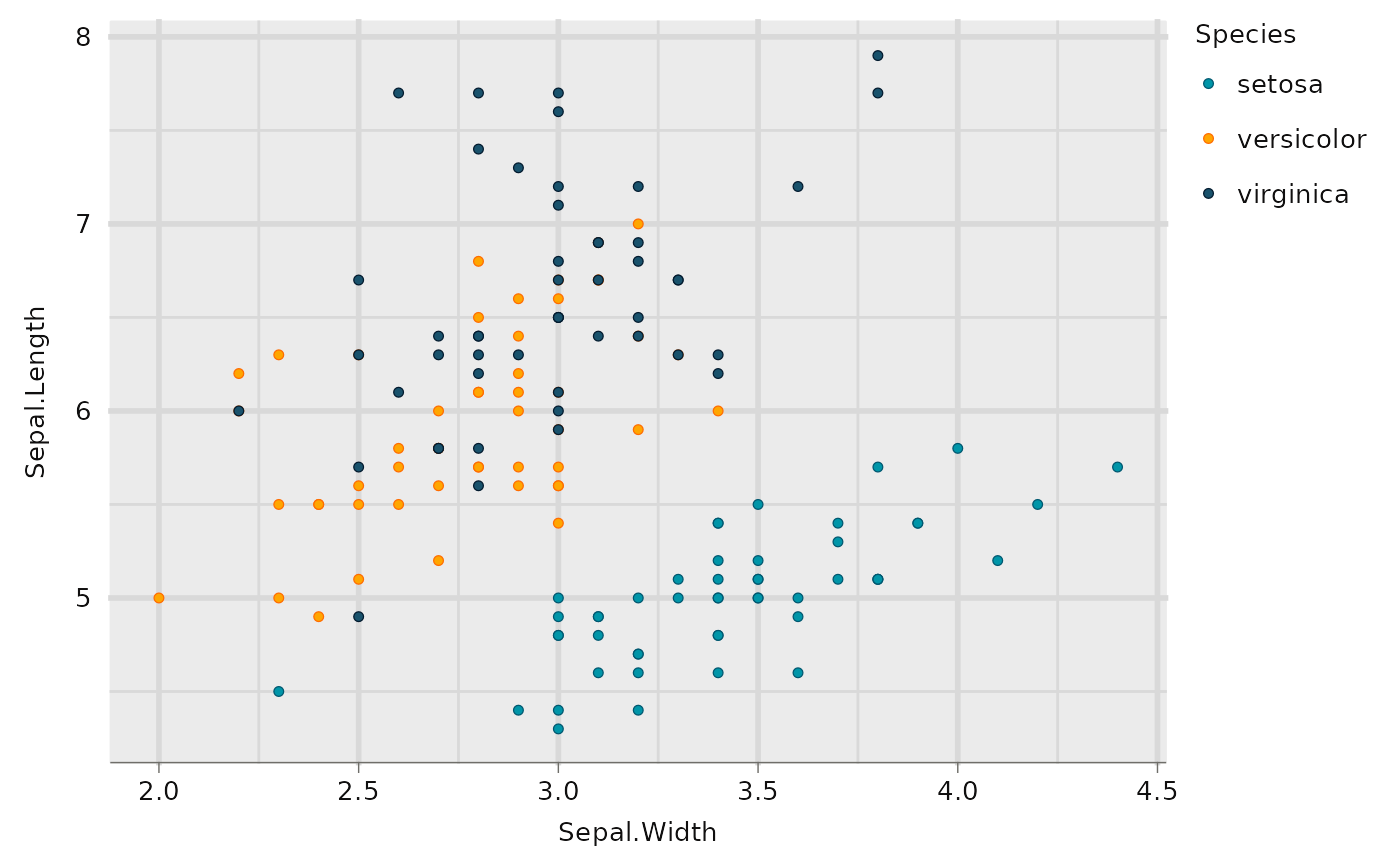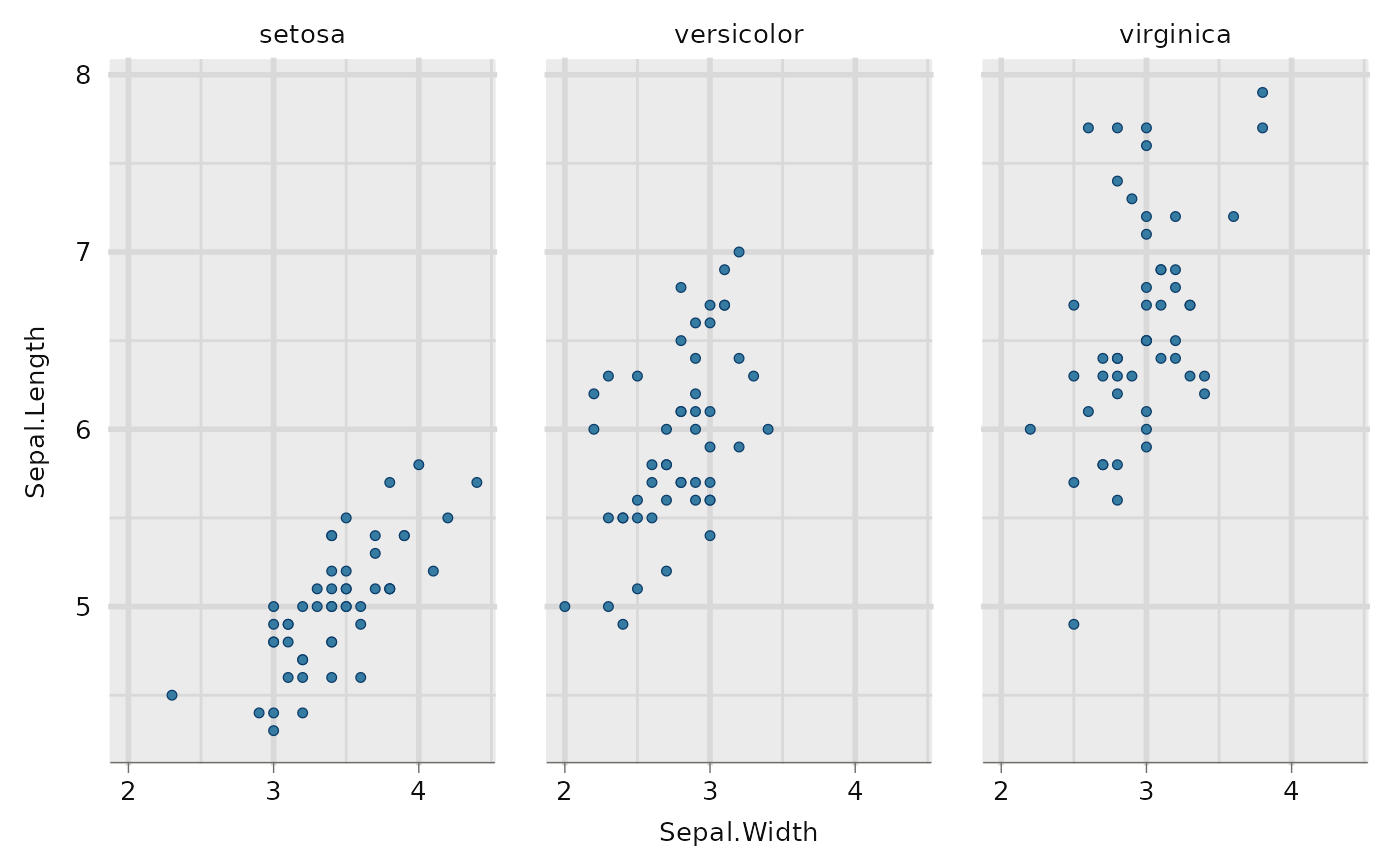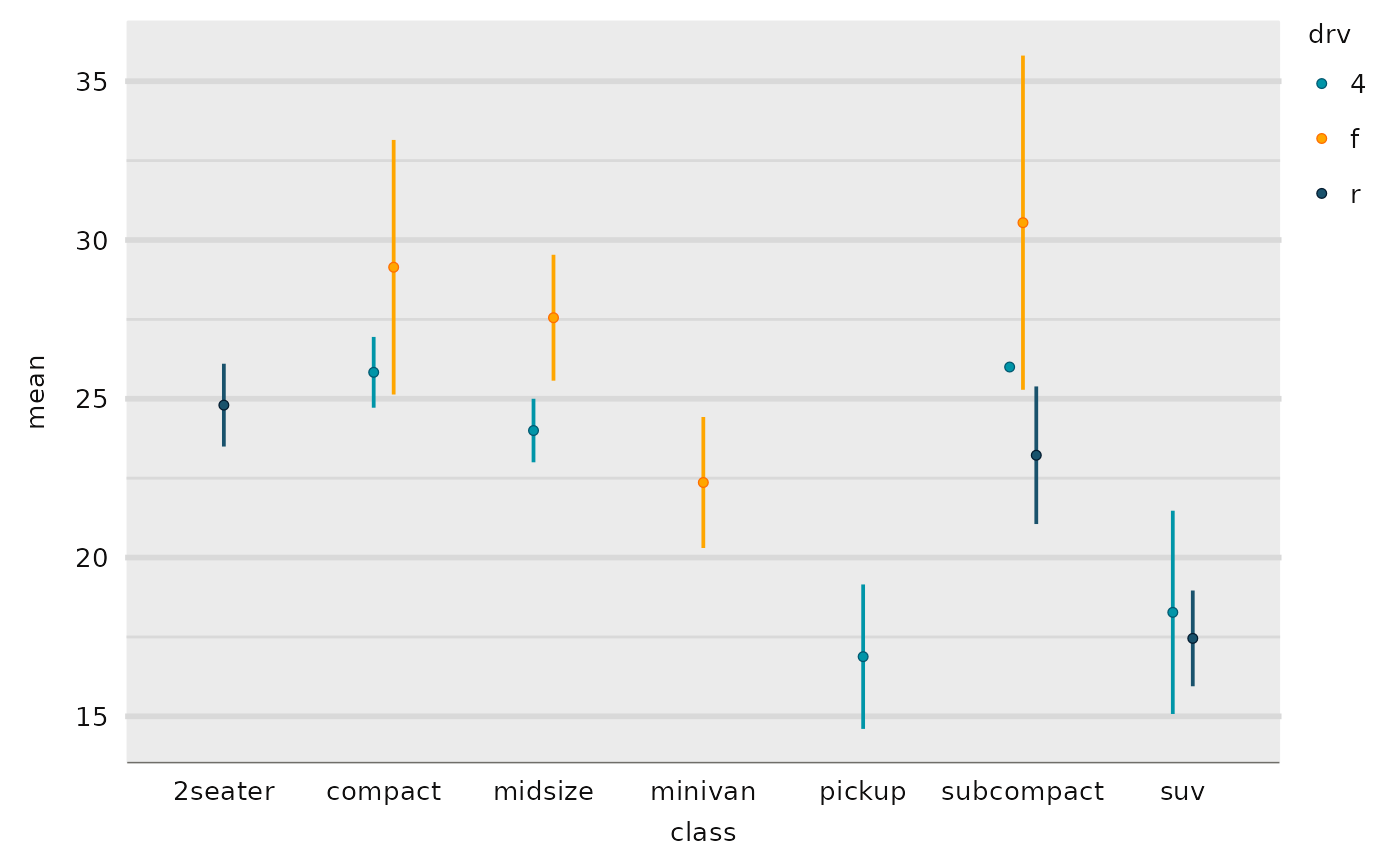Create a point ggplot with a wrapper around the ggplot2::geom_point function.

## Usage

gg_point(
data = NULL,
x = NULL,
y = NULL,
col = NULL,
facet = NULL,
facet2 = NULL,
group = NULL,
stat = "identity",
position = "identity",
clip = "on",
pal = NULL,
pal_na = "#7F7F7F",
alpha = 1,
...,
title = NULL,
subtitle = NULL,
x_breaks = NULL,
x_expand = NULL,
x_grid = NULL,
x_include = NULL,
x_labels = NULL,
x_limits = NULL,
x_sec_axis = ggplot2::waiver(),
x_title = NULL,
x_trans = "identity",
y_breaks = NULL,
y_expand = NULL,
y_grid = NULL,
y_include = NULL,
y_labels = NULL,
y_limits = NULL,
y_sec_axis = ggplot2::waiver(),
y_title = NULL,
y_trans = "identity",
col_breaks = NULL,
col_include = NULL,
col_labels = NULL,
col_legend_place = NULL,
col_legend_ncol = NULL,
col_legend_nrow = NULL,
col_legend_rev = FALSE,
col_limits = NULL,
col_rescale = NULL,
col_title = NULL,
col_trans = "identity",
facet_labels = NULL,
facet_ncol = NULL,
facet_nrow = NULL,
facet_scales = "fixed",
facet_space = "fixed",
facet_layout = NULL,
caption = NULL,
titles = snakecase::to_sentence_case,
theme = gg_theme()
)

## Arguments

data

A data frame or tibble.

x

Unquoted x aesthetic variable.

y

Unquoted y aesthetic variable.

col

Unquoted col and fill aesthetic variable.

facet

Unquoted facet aesthetic variable.

facet2

Unquoted second facet variable.

group

Unquoted group aesthetic variable.

stat

Statistical transformation. A character string (e.g. "identity").

position

Position adjustment. Either a character string (e.g."identity"), or a function (e.g. ggplot2::position_identity()).

clip

Whether to clip geometries outside of the panel. Either "on" or "off".

pal

Colours to use. A character vector of hex codes (or names).

pal_na

Colour to use for NA values. A character vector of a hex code (or name).

alpha

Opacity. A number between 0 and 1.

...

Other arguments passed to the ggplot2::geom_point function.

title

Title string.

subtitle

Subtitle string.

x_breaks

A function on the limits (e.g. scales::breaks_pretty()), or a vector of breaks.

x_expand

Padding to the limits with the ggplot2::expansion function, or a vector of length 2 (e.g. c(0, 0)).

x_grid

TRUE or FALSE for vertical x gridlines. NULL guesses based on the classes of the x and y.

x_include

For a numeric or date variable, any values that the scale should include (e.g. 0).

x_labels

A function that takes the breaks as inputs (e.g. scales::label_comma()), or a vector of labels.

x_limits

A vector of length 2 to determine the limits of the axis (and the zoom via the coord).

x_sec_axis

A secondary axis using the ggplot2::sec_axis or ggplot2::dup_axis function.

x_title

Axis title string. Defaults to converting to sentence case with spaces. Use "" for no title.

x_trans

For a numeric variable, a transformation object (e.g. "log10", "sqrt" or "reverse").

y_breaks

A function on the limits (e.g. scales::breaks_pretty()), or a vector of breaks.

y_expand

Padding to the limits with the ggplot2::expansion function, or a vector of length 2 (e.g. c(0, 0)).

y_grid

TRUE or FALSE of horizontal y gridlines. NULL guesses based on the classes of the x and y.

y_include

For a numeric or date variable, any values that the scale should include (e.g. 0).

y_labels

A function that takes the breaks as inputs (e.g. scales::label_comma()), or a vector of labels.

y_limits

A vector of length 2 to determine the limits of the axis (and the zoom via the coord).

y_sec_axis

A secondary axis using the ggplot2::sec_axis or ggplot2::dup_axis function.

y_title

Axis title string. Defaults to converting to sentence case with spaces. Use "" for no title.

y_trans

For a numeric variable, a transformation object (e.g. "log10", "sqrt" or "reverse").

col_breaks

A function on the limits (e.g. scales::breaks_pretty()), or a vector of breaks.

col_continuous

Type of colouring for a continuous variable. Either "gradient" or "steps". Defaults to "steps" - or just the first letter of these e.g. "g".

col_include

For a numeric or date variable, any values that the scale should include (e.g. 0).

col_labels

A function that takes the breaks as inputs (e.g. scales::label_comma()), or a vector of labels. Note this does not affect where col_intervals is not NULL.

col_legend_place

The place for the legend. Either "bottom", "right", "top" or "left" - or just the first letter of these e.g. "b".

col_legend_ncol

The number of columns for the legend elements.

col_legend_nrow

The number of rows for the legend elements.

col_legend_rev

Reverse the elements of the legend. Defaults to FALSE.

col_limits

A vector to determine the limits of the colour scale.

col_rescale

For a continuous col variable, a vector to rescale the pal non-linearly.

col_title

Legend title string. Defaults to converting to sentence case with spaces. Use "" for no title.

col_trans

For a numeric variable, a transformation object (e.g. "log10", "sqrt" or "reverse").

facet_labels

A function that takes the breaks as inputs (e.g. scales::label_comma()), or a named vector of labels (e.g. c("value" = "label", ...)).

facet_ncol

The number of columns of facets. Only applies to a facet layout of "wrap".

facet_nrow

The number of rows of facets. Only applies to a facet layout of "wrap".

facet_scales

Whether facet scales should be "fixed" across facets, "free" in both directions, or free in just one direction (i.e. "free_x" or "free_y"). Defaults to "fixed".

facet_space

Whether facet space should be "fixed" across facets, "free" to be proportional in both directions, or free to be proportional in just one direction (i.e. "free_x" or "free_y"). Defaults to "fixed". Only applies where the facet layout is "grid" and facet scales are not "fixed".

facet_layout

Whether the layout is to be "wrap" or "grid". If NULL and a single facet (or facet2) argument is provided, then defaults to "wrap". If NULL and both facet and facet2 arguments are provided, defaults to "grid".

caption

Caption title string.

titles

A function to format the x, y and col titles. Defaults to snakecase::to_sentence_case.

theme

A ggplot2 theme.

A ggplot object.

## Examples

library(ggplot2)

gg_point(mtcars, x = wt, y = mpg)gg_point(mtcars, x = wt, y = mpg, col = cyl)mtcars %>%
dplyr::mutate(cyl = factor(cyl)) %>%
gg_point(x = wt, y = mpg, col = cyl, size = 1)gg_point(diamonds, x = carat, y = price, alpha = 0.01)